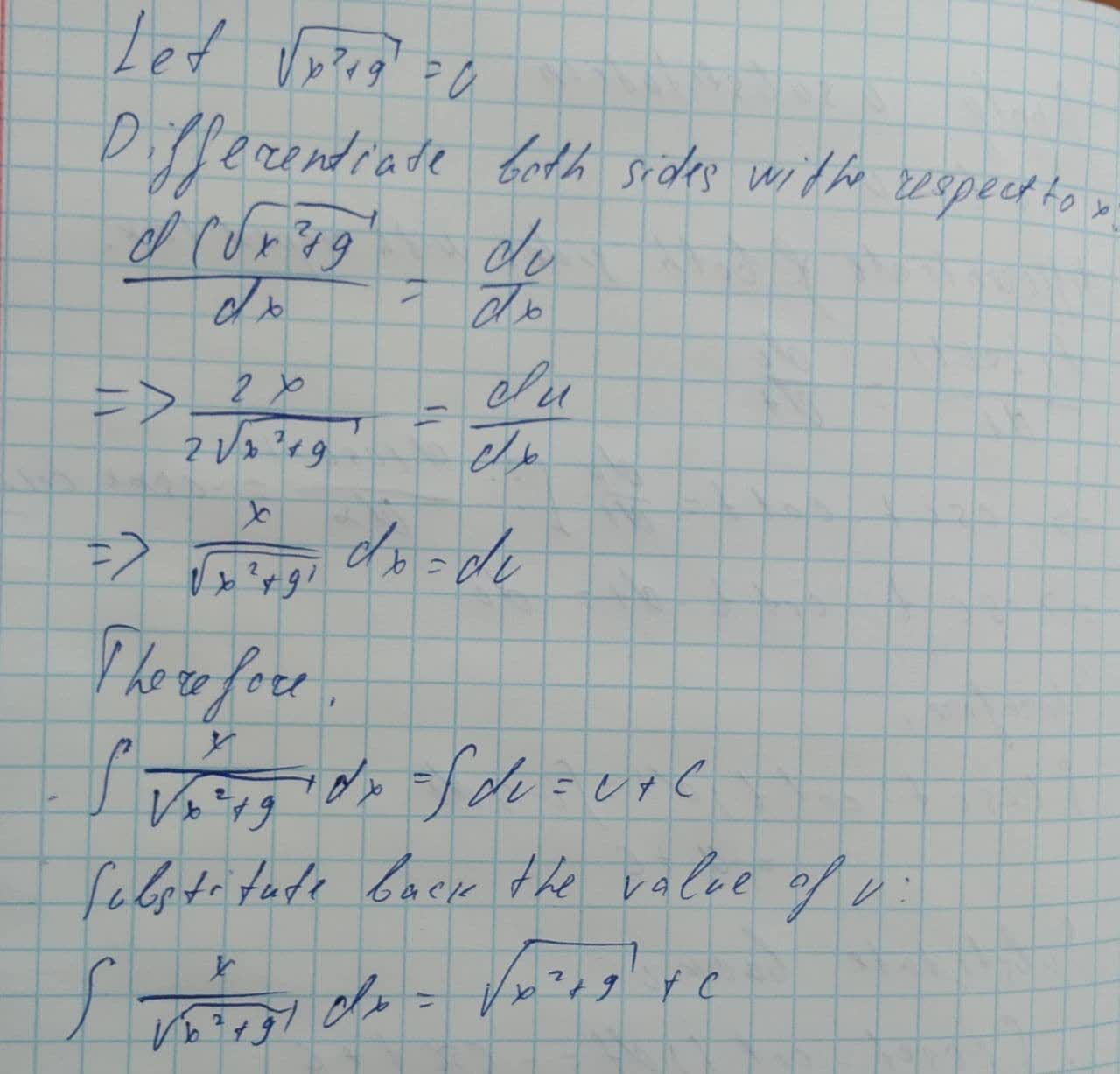Question# Evaluate the indefinite integral. \int \frac{x}{\sqrt{x^{2}+9}}dx

Applications of integrals
ANSWEREDEvaluate the indefinite integral.
$$\displaystyle\int{\frac{{{x}}}{{\sqrt{{{x}^{{{2}}}+{9}}}}}}{\left.{d}{x}\right.}$$